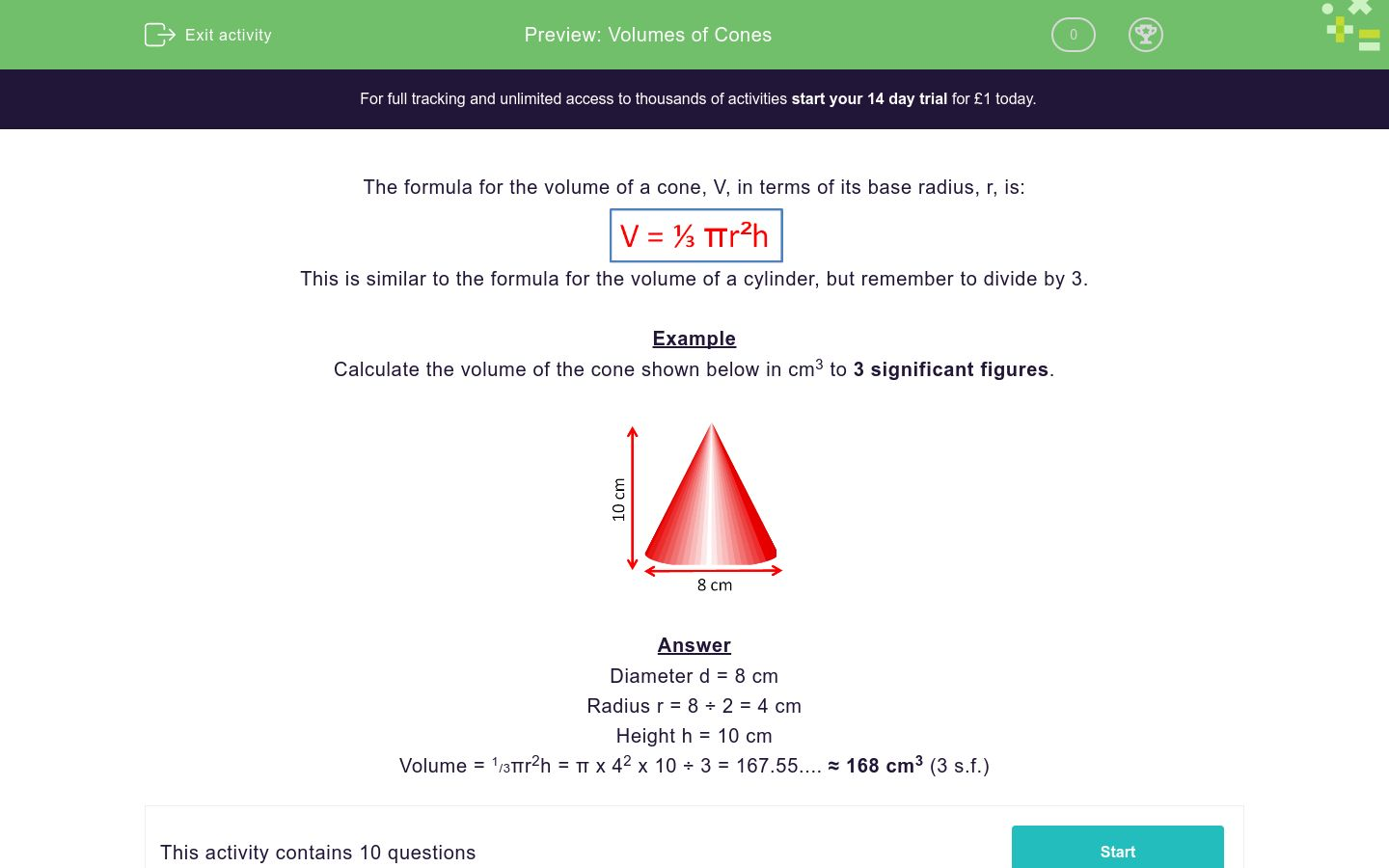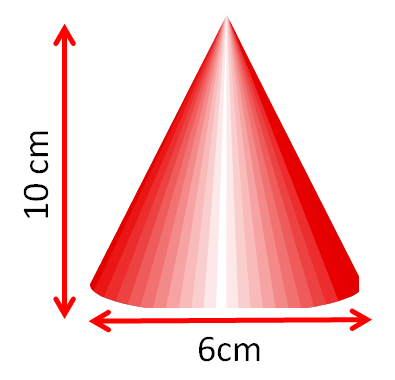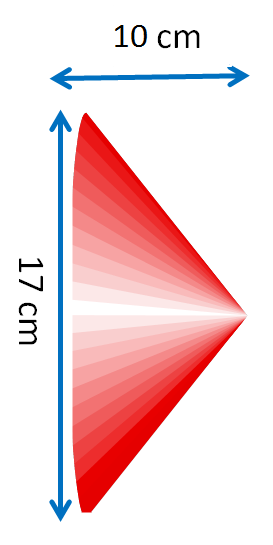Volumes of Cones

In this worksheet, students calculate the volume of cones, given their height and base diameter.Key stage:  KS 3

Curriculum topic:  Geometry and Measures

Curriculum subtopic:  Use 3D Shape Knowledge to Solve Problems

Difficulty level:QUESTION 1 of 10

The formula for the volume of a cone, V, in terms of its base radius, r, is:This is similar to the formula for the volume of a cylinder, but remember to divide by 3.

Example

Calculate the volume of the cone shown below in cm3 to 3 significant figures.Diameter d = 8 cm

Radius r = 8 ÷ 2 = 4 cm

Height h = 10 cm

Volume = 1/3πr2h = π x 42 x 10 ÷ 3 = 167.55.... ≈ 168 cm3 (3 s.f.)

Calculate the volume of the cone shown below in cm3 to 3 sig. figs.

Just write the number.Calculate the volume of the cone shown below in cm3 to 3 sig. figs.

Just write the number.Calculate the volume of the cone shown below in cm3 to 3 sig. figs.

Just write the number.Calculate the volume of the cone shown below in cm3 to 3 sig. figs.

Just write the number.Calculate the volume of the cone shown below in cm3 to 3 sig. figs.

Just write the number.Calculate the volume of the cone shown below in cm3 to 3 sig. figs.

Just write the number.Calculate the volume of the cone shown below in cm3 to 3 sig. figs.

Just write the number.Calculate the volume of the cone shown below in cm3 to 3 sig. figs.

Just write the number.Calculate the volume of the cone shown below in cm3 to 3 sig. figs.

Just write the number.Calculate the volume of the cone shown below in cm3 to 3 sig. figs.

Just write the number.• Question 1

Calculate the volume of the cone shown below in cm3 to 3 sig. figs.

Just write the number.94.2
EDDIE SAYS
Vol = π × 3² × 10 ÷ 3
• Question 2

Calculate the volume of the cone shown below in cm3 to 3 sig. figs.

Just write the number.104
EDDIE SAYS
Vol = π × 3² × 11 ÷ 3
• Question 3

Calculate the volume of the cone shown below in cm3 to 3 sig. figs.

Just write the number.72.0
EDDIE SAYS
Vol = π × 2.5² × 11 ÷ 3
• Question 4

Calculate the volume of the cone shown below in cm3 to 3 sig. figs.

Just write the number.605
EDDIE SAYS
Vol = π × 8.5² × 8 ÷ 3
• Question 5

Calculate the volume of the cone shown below in cm3 to 3 sig. figs.

Just write the number.85.1
EDDIE SAYS
Vol = π × 2.5² × 13 ÷ 3
• Question 6

Calculate the volume of the cone shown below in cm3 to 3 sig. figs.

Just write the number.128
EDDIE SAYS
Vol = π × 3.5² × 10 ÷ 3
• Question 7

Calculate the volume of the cone shown below in cm3 to 3 sig. figs.

Just write the number.37.7
EDDIE SAYS
Vol = π × 2² × 9 ÷ 3
• Question 8

Calculate the volume of the cone shown below in cm3 to 3 sig. figs.

Just write the number.18.8
EDDIE SAYS
Vol = π × 1.5² × 8 ÷ 3
• Question 9

Calculate the volume of the cone shown below in cm3 to 3 sig. figs.

Just write the number.757
EDDIE SAYS
Vol = π × 8.5² × 10 ÷ 3
• Question 10

Calculate the volume of the cone shown below in cm3 to 3 sig. figs.

Just write the number.205000
EDDIE SAYS
Vol = π × 62.5² × 50 ÷ 3
---- OR ----

Sign up for a £1 trial so you can track and measure your child's progress on this activity.

What is EdPlace?

We're your National Curriculum aligned online education content provider helping each child succeed in English, maths and science from year 1 to GCSE. With an EdPlace account you’ll be able to track and measure progress, helping each child achieve their best. We build confidence and attainment by personalising each child’s learning at a level that suits them.